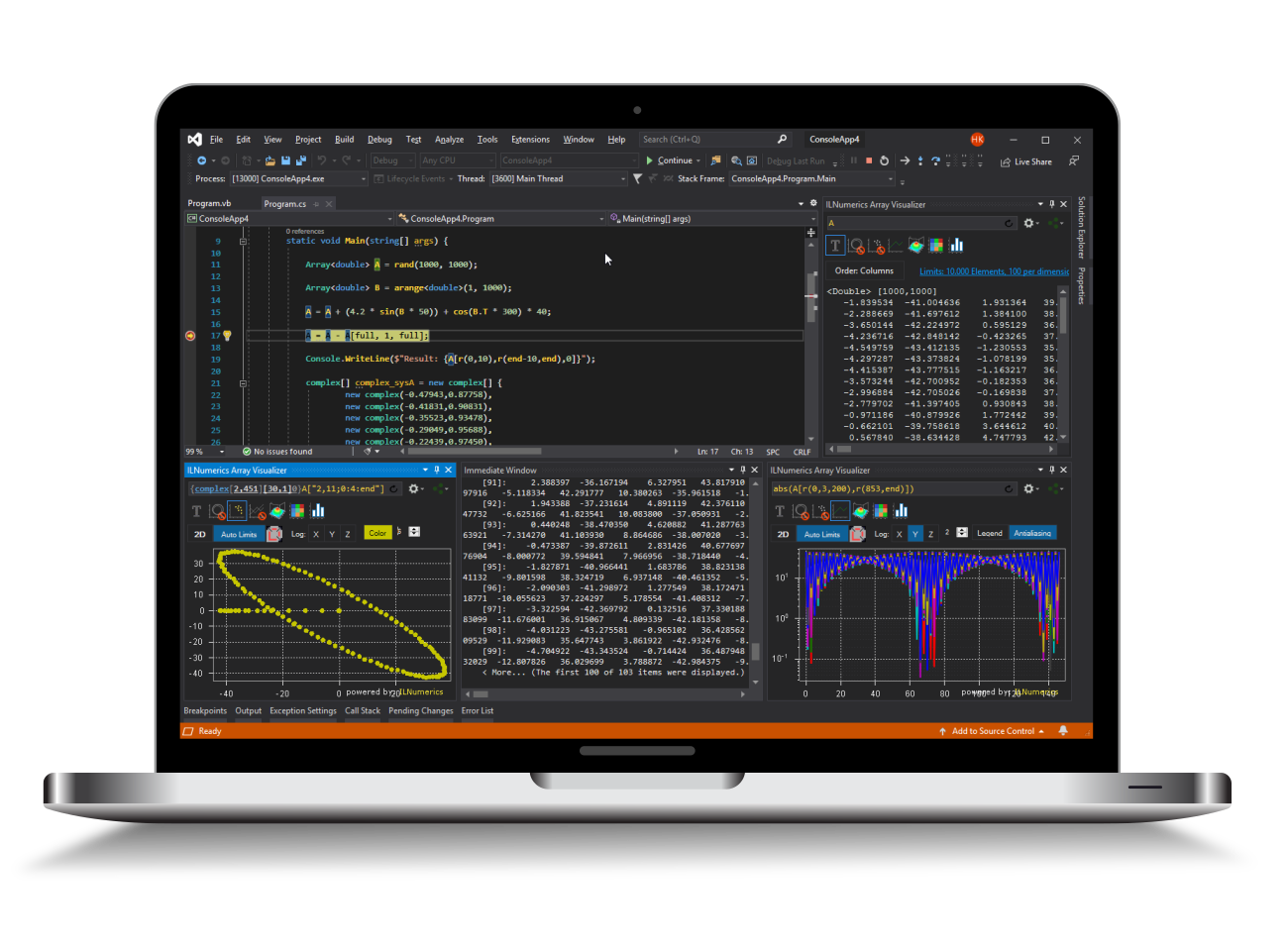Industrial Data Science
in C# and .NET:
Simple. Fast. Reliable.ILNumerics - Technical Computing

Modern High Performance Tools for Technical

Computing and Visualization in Industry and Science

tgt

# Systems of linear equations

In general, linear equation systems are equations in the form: A x=b, where

• A is matrix [nxm],
• x is vector [mx1] and
• b is called the 'right hand side' vector of size [nx1].

The matrix A and the right hand side b are given, so we are seeking for an x which solves the equation by means of rounding errors.

Whether such an x exists, depends on the shape and content of A. The solution x theoretically gets determined by inversion of A, as x = A-1 b.

Once we have the inverse of A, solutions xi for any other right hand sides bi can be easyly computed by a simple matrix multiplication: xi = A-1bi. Just as well we could rewrite the general equation definition, to include any number of right hand sides: A X=B, where

• A is matrix of size [n x m], X is matrix [m x q] and B now the 'right hand side' matrix [n x q].

Here the columns of B are the individual right hand sides for each equation system, the columns of X hold each solution vector.

### Solving systems of linear equations

Since for larger matrices inverting A would be the most expensive way to solve such a system of equations, other methods exists. Which method to choose depends on the structure of A. ILNumerics automatically determines the best method to use. Therefore the structure for given matrix A is determined first and the solution is computed by one of the following methods. All linear equations are solved by use of the function ILMath.linsolve. The usage is the same for all methods. Therefore only one example was given for the first method.

If A is square (m == n) and an upper or lower triangular matrix, the system will directly be solved via backward- or forward substitution in the LAPACK function ?trtrs.

If A is found to be square and symmetric or hermitian, A will be decomposed into a triangular equation system using cholesky factorization via LAPACK. The system is than solved using performant LAPACK routines from the method above. If during the cholesky factorization A was found to be not positive definite - the cholesky factorization is canceled and the next method is tested.

If A is square only, it will be decomposed into upper and lower triangular matrices using LU decomposition and LAPACK. The triangular system is than solved using performant LAPACK routines from the first method.

Otherwise, if A is of size [q x n] and q != n, the system is solved using QR decomposition. This can be imagined as kind of an optimization problem. The solution is one, which solves the equations best - i.e. the error will be smallest. A may be rank deficient. The solution is computed by use of the LAPACK routine '?gelsy'.

Again, since the method for solving is determined automatically, calling ILMath.linsolve(A,B) with Matrix A and right hand side b is sufficient for solution.

### Optimizing solver performance

If one must count on performance, it is possible to skip the tests for special matrix structures. So, if it is clear the matrix A is symmetric, positive definite (because the underlying physical modell prooves this, lets say - it comes from a partial differential equation problem, modelled by finite differences, f.e.), one can skip the test for symmetry and others. Therefore a structure MatrixProperties is used and given as third argument to ILMath.linsolve.

The structure MatrixProperties holds a number of special matrix structures which can get combined into arbitrary combinations. Here comes the collection of possible flags:

However, using those properties is optional, since, if no properties are specified, ILNumerics will automatically determine the structure.

The following example demonstrates using MatrixProperties for an symmetric, positiv definite matrix. Therefore the test on symmetry is saved.

The MatrixProperties struct is given by reference, since it may be altered inside linsolve. This happens, if linsolve finds some properties not set correctly.